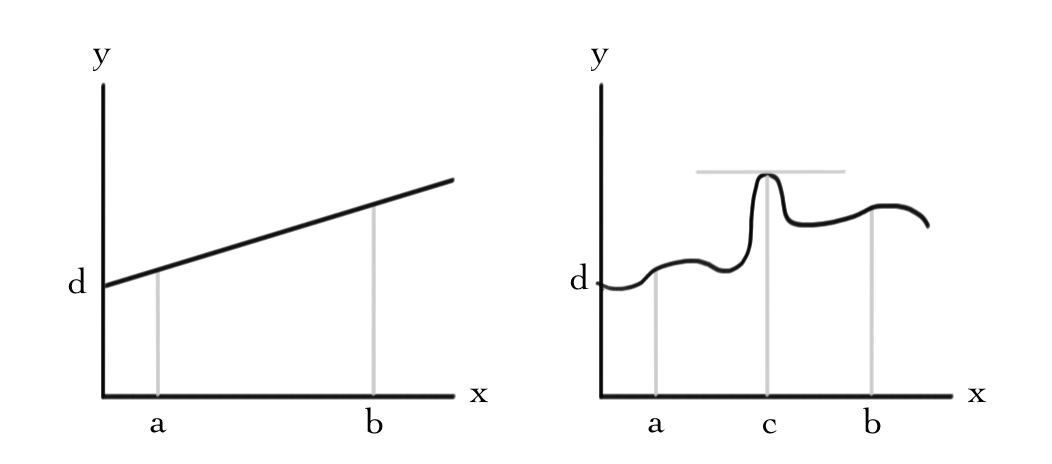$$\newcommand{\id}{\mathrm{id}}$$ $$\newcommand{\Span}{\mathrm{span}}$$ $$\newcommand{\kernel}{\mathrm{null}\,}$$ $$\newcommand{\range}{\mathrm{range}\,}$$ $$\newcommand{\RealPart}{\mathrm{Re}}$$ $$\newcommand{\ImaginaryPart}{\mathrm{Im}}$$ $$\newcommand{\Argument}{\mathrm{Arg}}$$ $$\newcommand{\norm}{\| #1 \|}$$ $$\newcommand{\inner}{\langle #1, #2 \rangle}$$ $$\newcommand{\Span}{\mathrm{span}}$$

# 3.2: Graphical Solutions

$$\newcommand{\vecs}{\overset { \rightharpoonup} {\mathbf{#1}} }$$ $$\newcommand{\vecd}{\overset{-\!-\!\rightharpoonup}{\vphantom{a}\smash {#1}}}$$$$\newcommand{\id}{\mathrm{id}}$$ $$\newcommand{\Span}{\mathrm{span}}$$ $$\newcommand{\kernel}{\mathrm{null}\,}$$ $$\newcommand{\range}{\mathrm{range}\,}$$ $$\newcommand{\RealPart}{\mathrm{Re}}$$ $$\newcommand{\ImaginaryPart}{\mathrm{Im}}$$ $$\newcommand{\Argument}{\mathrm{Arg}}$$ $$\newcommand{\norm}{\| #1 \|}$$ $$\newcommand{\inner}{\langle #1, #2 \rangle}$$ $$\newcommand{\Span}{\mathrm{span}}$$ $$\newcommand{\id}{\mathrm{id}}$$ $$\newcommand{\Span}{\mathrm{span}}$$ $$\newcommand{\kernel}{\mathrm{null}\,}$$ $$\newcommand{\range}{\mathrm{range}\,}$$ $$\newcommand{\RealPart}{\mathrm{Re}}$$ $$\newcommand{\ImaginaryPart}{\mathrm{Im}}$$ $$\newcommand{\Argument}{\mathrm{Arg}}$$ $$\newcommand{\norm}{\| #1 \|}$$ $$\newcommand{\inner}{\langle #1, #2 \rangle}$$ $$\newcommand{\Span}{\mathrm{span}}$$

Before giving a more general algorithm for handling this problem and problems like it, we note that when the number of variables is small (preferably 2), a graphical technique can be used.

Inequalities, such as the four given in Pablo's problem, are often called $$\textit {constraints}$$, and values of the variables that satisfy these constraints comprise the so-called $$\textit {feasible region}$$. Since there are only two variables, this is easy to plot:

Example 35: Constraints and feasible region

Pablo's constraints are

\begin{eqnarray*}
&x\geq 5&\\
&y\geq 7&\\[2mm]
&15\leq x+y\leq25\, .&
\end{eqnarray*}

Plotted in the $$(x,y)$$ plane, this gives:You might be able to see the solution to Pablo's problem already. Oranges are very sugary, so they should be kept low, thus $$y=7$$. Also, the less fruit the better, so the answer had better lie on the line $$x+y=15$$. Hence, the answer must be at the vertex $$(8,7)$$. Actually this is a general feature of linear programming problems, the optimal answer must lie at a vertex of the feasible region. Rather than prove this, lets look at a plot of the linear function $$s(x,y)=5x+10y$$.

Example 36: The sugar function

Plotting the sugar function requires three dimensions:The plot of a linear function of two variables is a plane through the origin. Restricting the variables to the feasible region gives some lamina in 3-space. Since the function we want to optimize is linear (and assumedly non-zero), if we pick a point in the middle of this lamina, we can always increase/decrease the function by moving out to an edge and, in turn, along that edge to a corner. Applying this to the above picture, we see that Pablo's best option is 110 grams of sugar a week, in the form of 8 apples and 7 oranges.

It is worthwhile to contrast the optimization problem for a linear function with the non-linear case you may have seen in calculus courses:Here we have plotted the curve $$f(x)=d$$ in the case where the function $$f$$ is linear and non-linear. To optimize $$f$$ in the interval $$[a,b]$$, for the linear case we just need to compute and compare the values $$f(a)$$ and $$f(b)$$. In contrast, for non-linear functions it is necessary to also compute the derivative $$\frac{\mathrm{d} f}{\mathrm{d} x}$$ to study whether there are extrema inside the interval.